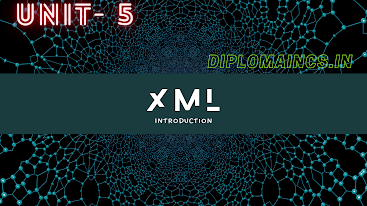## 6/17/22

### Computer Graphics Question 2021

Computer Graphics Question

Sub Code: - 1618604

2021(Even)

Time : 3Hrs

Semester  VI(New)/CSE

Computer Graphics

Full Marks : 70

Pass marks : 28

Group A

Choose the most suitable answer from the following options: -   (1*20=20)

(i) What is the fill form of GIF

(a) Graphical Interface Format

(b) Graphical Interchange Format

(c)  Graphical Image Format

(d) Graphical Image File

(ii) In LCD the refresh rate of the screen is

(a)     100 frame/sec

(b)    80 frame/sec

(c)     30 frame/sec

(d)    60 frame/sec

(iii) The two dimensional scaling equation in the matrix form is

(a)         P' = P + T

(b)         P' =P * R

(c)          P' =S * P

(d)         P' =R + S

(iv) Which of the following is not a type of orthographic projection?

(a)         Top Projection

(b)         Side Projection

(c)          Cavalier Projection

(d)         Axonometric projection

(v) Which of the following is not a transformation technique?

(a) Scaling

(b) Shearing

(c) Translation

(d) Revolution

(vi) If scaling parameter towards x-axis is 2 and towards y-axis is 3 then after scaling new coordinate of point (-1,2) is

(a)         (2, 6)

(b)         (-3, 4)

(c)          (-2, 6)

(d)         (2, -6)

(vii) What is the full form of TIFF?

(a)         Tagged image full format

(b)          Translated image file format

(c)           Translated image full format

(d)         Tagged image file format

(viii) Which of the following is not a input device?

(a)         Joystick

(b)         Light pen

(c)          Key board

(d)         Speaker

(ix) What is the full form of DDA algorithm?

(a)          Digital Difference Analyzer

(b)         Direct Differential Analyzer

(c)           Data Differential Analyzer

(d)         Digital Differential Analyzer

(x) If the scaling factor values Sx and Sy are assigned to the same value them

(a)  Uniform rotation is produced

(b) Uniform Scaling is produced

(c) Scaling cannot be done

(d) Both A and B

(xi) On raster scan, lines are plotted with

(a)         Points

(b)         Dots

(c)          Pixels

(d)         None of the above

(xii) The transformation which is used to change the size of an object is

(a)         Rotation

(b)          Scaling

(c)          Shearing

(d)         Translation

(xiii) The original coordinate of the point in polar coordinate are

(a)         X' = r cos (θ + ϕ) and Y' = r cos (θ + ϕ)

(b)         X'  = r cos (θ + ϕ) and Y' = r sin (θ + ϕ)

(c)          X'  = r cos (ϕ - θ) and Y' = r cos (ϕ - θ)

(d)         X'  = r cos (ϕ + θ) and Y' = r sin (ϕ - θ)

(xiv) To change the position of a circle or ellipse we translate

(a)          Center coordinates and redraw the figure in new location

(b)         Outline Coordinates

(c)           Center Coordinates

(d)         All of the mentioned

(xv) Which of the following is not a type of clipping?

(a)         Area clipping

(b)         Curve clipping

(c)          Pixel clipping

(d)         Text clipping

(xvi) In Breserham’s line drawing algorithm if the distance d1<d2 then decision parameter P­k is

(a)         Positive

(b)         Negative

(c)          Equal

(d)         Zero

(xvii) The total number of regions in Cohen Sutherland algorithm is

(a)         6

(b)         3

(c)          9

(d)          7

(xviii) Orthographic projection is also known as

(a)         Single view projection

(b)         Multi – view projection

(c)          Two view projection

(d)         All of the above

(xix) The number of pixels stored in the frame buffer of a graphics system is called

(a)         Depth

(b)         Resolution

(c)          Sensitivity

(d)         Susceptivity

(xx) In a 2-D translation, a point (x, y) can move to the new position (x, y) by using the equation

(a)         x= x + dx and y = y + dx

(b)         x= x + dy and y = y - dx

(c)          x= x - dx and y = y - dy

(d)         x= x + dx and y = y + dy

Group:-"B"

Answer all Five Questions: -                         (5*4=20)

2.  What is Computer Graphics? Explain various applications of Computer Graphics.

OR

Explain two dimensional transformation. Right name of different transformation techniques

3. Explain parallel projection and perspective projection

OR

What do you mean by animation? Explain types of animation system.

4. Explain Cohen Sutherland algorithm.

OR

What do you mean by clipping? Explain different clipping techniques

5. Explain DDA line drawing algorithm with example

OR

Differentiate between Random scan and Rashtra scan?

6. Write three any different display devices. Explain functioning of LCD and LED display

OR

Explain feeling techniques in computer graphics

Group:- "C"

Answer all Five Questions: -                         (5*6=30)

7. Write algorithms for DDA line drawing techniques. Calculate the points between the starting point (9, 18) and the ending point (14, 22) using DDA line drawing algorithm

OR

Explain properties of Bezier curve and B-spline curve. Explain the advantage of B-line curve over Bezier curve.

8. Explain Bresenham's circle drawing algorithm. Plot 4 points of circle using bresenham's algorithm with radius of 10 unit and centre (50, 50).

OR

Explain various three-dimensional transformation with examples.

9. Explain parallel projection. Explain different type of parallel projection.

OR

Explain CRT with diagram. Right any three input and output devices used in computer graphics.

10. Write short notes on any two of the following: -

a.   Bresenham’s line drawing algorithm

b.   Perspective projection

c.    Polygon clipping

OR

Explain Windows and viewports. Write steps for Cohen Sutherland algorithm.

11. Explain two-dimensional transformation techniques. Explain shearing and scaling technique in detail.

OR

Explain perspective projection. What do you mean by hidden surface removal and back surface removal.